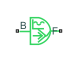# Sinusoidal Torque Source

Produce sinusoidal torque

•Libraries:
Simscape / Driveline / Sources

## Description

The Sinusoidal Torque Source block produces sinusoidal torque with a constant offset. A positive torque value generates torque acting from port B to port F.

The defining equation for the sinusoidal torque that the block generates is

`$T={T}_{o}+A*\mathrm{sin}\left(2\pi ft+\phi \right),$`

where:

• T is the output torque.

• To is the offset torque of the output.

• A is the peak amplitude of the sinusoid.

• f is frequency of the sinusoid in Hz.

• t is time.

• φ is phase shift of the sinusoid.

## Ports

### Conserving

expand all

Mechanical rotational conserving port associated with the base interface.

Mechanical rotational conserving port associated with the follower interface.

## Parameters

expand all

Average value that the sinusoidal output torque oscillates about.

Peak amplitude of the sinusoidal output torque.

Peak amplitude of the sinusoidal output torque.

Frequency of the sinusoidal output torque. The frequency must be greater than or equal to 0.

## Version History

Introduced in R2016b